## Bayes' Formula

Letandbe Sets. Conditional Probability requires that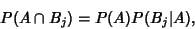(1)

wheredenotes Intersection (and''), and also that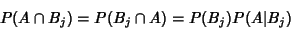(2)

and(3)

Since (2) and (3) must be equal,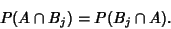(4)

From (2) and (3),(5)

Equating (5) with (2) gives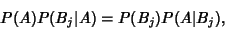(6)

so(7)

Now, let(8)

sois an event in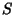and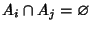for, then(9)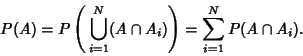(10)

From (5), this becomes(11)

so(12)